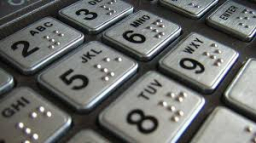# Probability 72324

We used the digits 2, 3, 4, 5, and 7 when entering the PIN code, and we only used each digit once. What is the probability that someone will guess our PIN code on the first try?

p =  0.01 %

### Step-by-step explanation:Did you find an error or inaccuracy? Feel free to write us. Thank you!

Tips for related online calculators
Need help calculating sum, simplifying, or multiplying fractions? Try our fraction calculator.